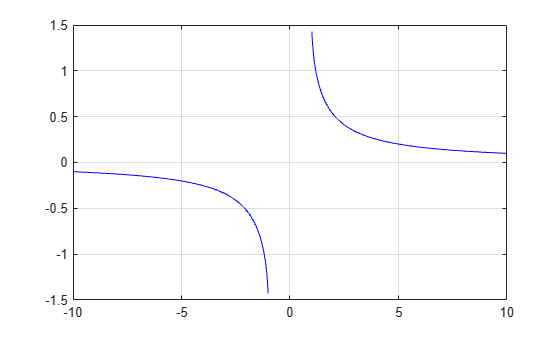# acsc

## Syntax

``Y = acsc(X)``

## Description

example

````Y = acsc(X)` returns the Inverse Cosecant (csc-1) of the elements of `X` in radians. The function accepts both real and complex inputs. For real values of `X` in the intervals [-∞, -1] and [1, ∞], `acsc(X)` returns real values in the interval [-π/2, π/2].For real values of `X` in the interval (-1, 1) and for complex values of `X`, `acsc(X)` returns complex values. ```

## Examples

collapse all

Find the inverse cosecant of a value.

`acsc(3)`
```ans = 0.3398 ```

Find the inverse cosecant of the elements of vector `x`. The `acsc` function acts on `x` element-wise.

```x = [0.5i 1+3i -2.2+i]; Y = acsc(x)```
```Y = 1×3 complex 0.0000 - 1.4436i 0.0959 - 0.2970i -0.3795 - 0.1833i ```

Plot the inverse cosecant function over the intervals $-10\le x<-1$ and $1.

```x1 = -10:0.01:-1.01; x2 = 1.01:0.01:10; plot(x1,acsc(x1),'b') hold on plot(x2,acsc(x2),'b') grid on```## Input Arguments

collapse all

Cosecant of angle, specified as a scalar, vector, matrix, multidimensional array, table, or timetable. The `acsc` operation is element-wise when `X` is nonscalar.

Data Types: `single` | `double` | `table` | `timetable`
Complex Number Support: Yes

collapse all

### Inverse Cosecant

The inverse cosecant is defined as

`${\mathrm{csc}}^{-1}\left(z\right)={\mathrm{sin}}^{-1}\left(\frac{1}{z}\right).$`

## Version History

Introduced in R2006a

expand all